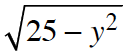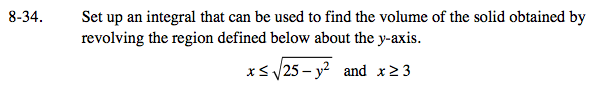### Home > CALC > Chapter 8 > Lesson 8.1.3 > Problem8-34

8-34.
1. Set up an integral that can be used to find the volume of the solid obtained by revolving the region defined below about the y-axis. Homework Help ✎

2. xand x ≥ 3Notice that BOTH functions are in x = ______ form. Some students find it easier to visualize y = ________ form. Feel free to 'switch the x and y' in both functions. As long as you switch ALL variables in both equations, the area and volume will be the same either way.

But you do not have to switch the variables. The semi-circle shape should have been easy to recognize!

Visualize the solid that will be generated after rotating this flag about the y-axis. It will look a bit like a donut.

Since the resultant solid has a hole in the center, use the Washer Method:

where f(y) is the graph with the longer radius and g(y) is the graph with the shorter radius.

Remember that the bounds are y-values, not x-values. That is, unless you switched the x and y as an initial step.